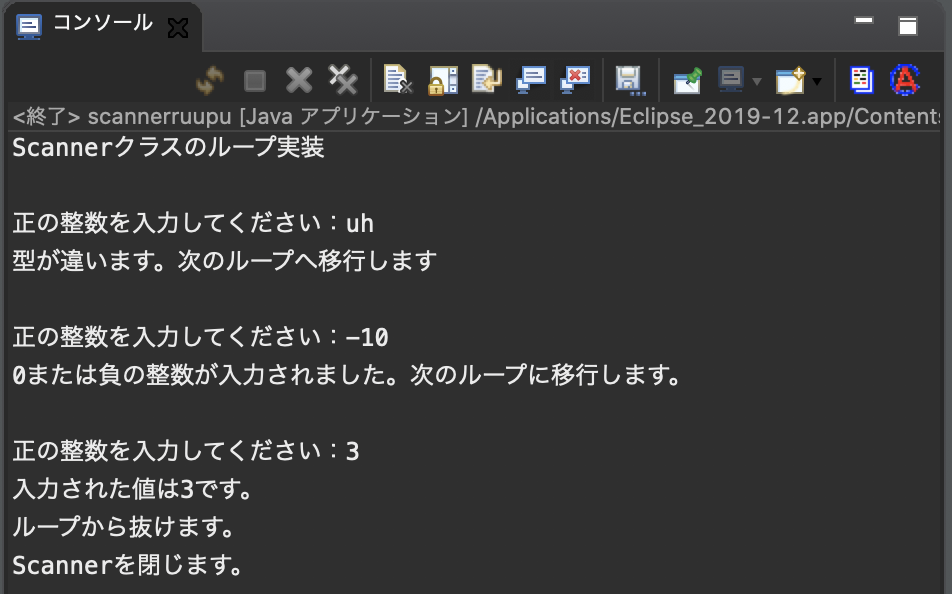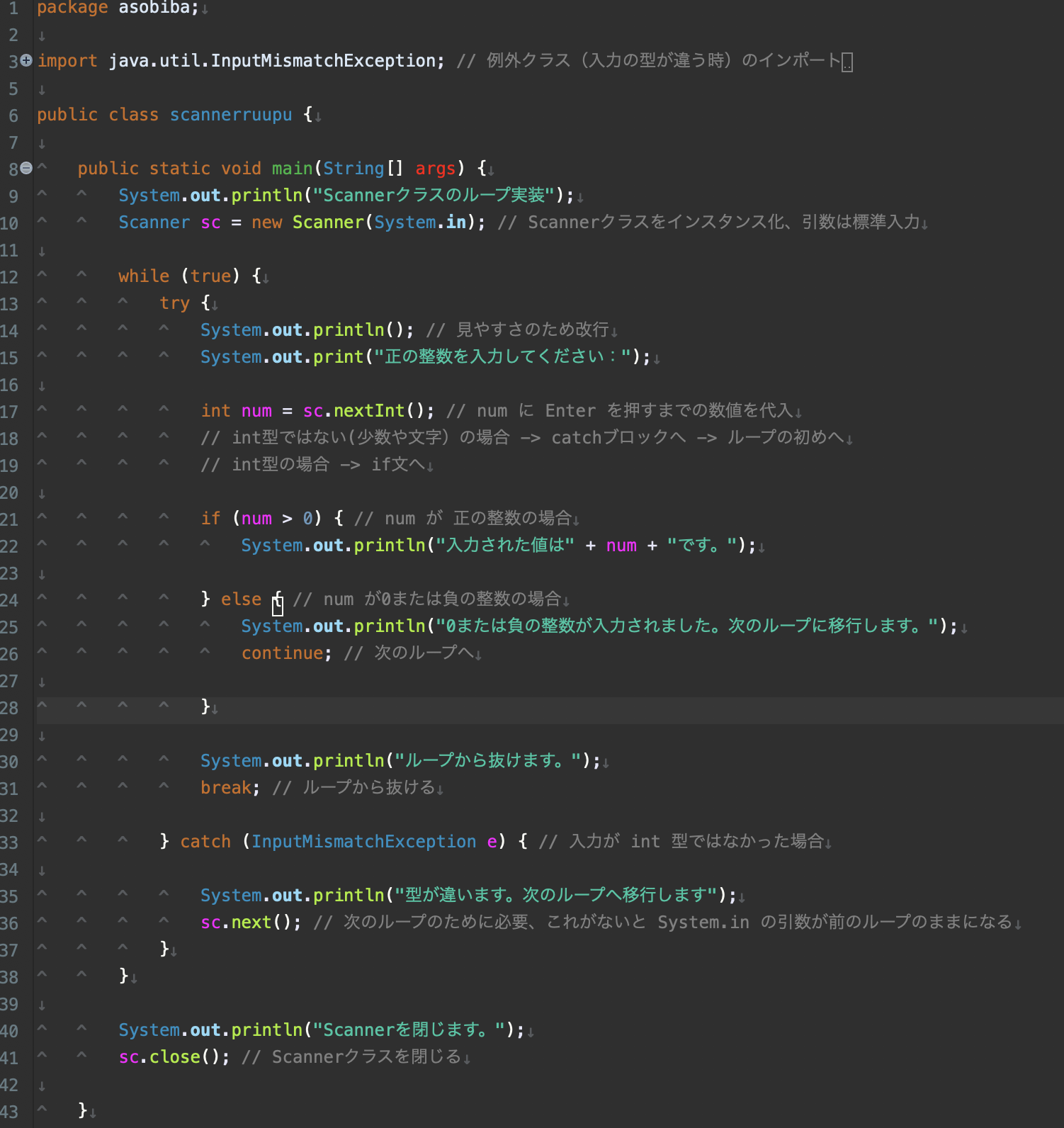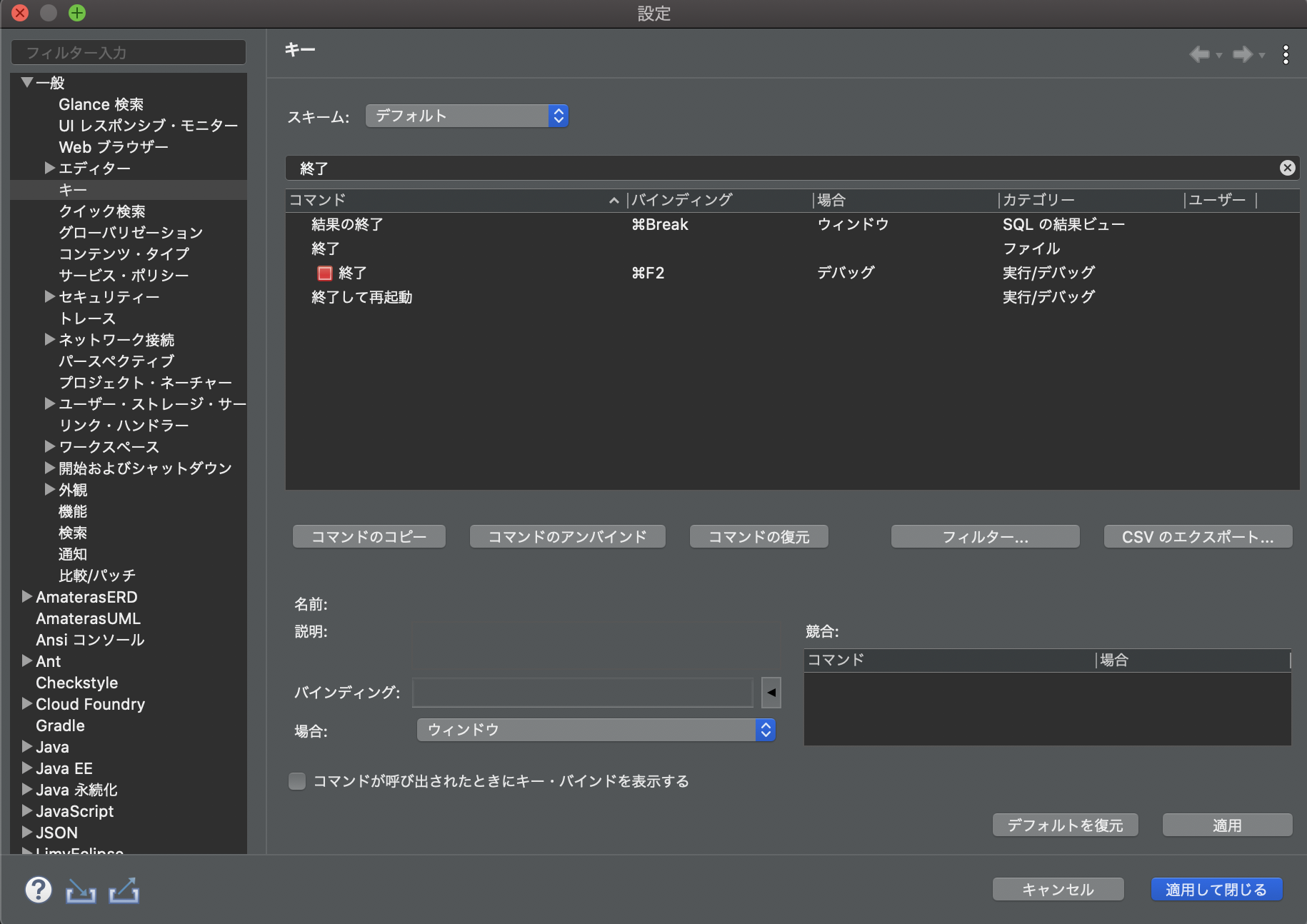# Scanner Infinite loop until normal input

## Last time

Last time used the try-catch syntax to implement the processing when the exception class is used.

This time, I will explain how to loop when the input is different using the if statement, try-catch syntax, and while statement considering all the input patterns.

Let's have an example!

## example

-Outputs a numerical value only when the input is a positive integer value, and returns to the input in other cases.

consoleFirst of all, please be aware that 0 is not a positive integer. (Contents of junior high school students) Line breaks for each loop are made for readability. I tried to make it easier to follow the movement of the code by outputting the processing notification statement.

** Pay particular attention to `break;` `` and `` continue;`. ** ** The detailed explanation of commenting out is in the back, so please read it for the time being even if you do not understand (^^ ;;

codeConsole screen (again)### Overall structure

• The try-catch syntax is looped with a while statement. The reason why the process that does not cause an error is put in the try block is that the scope (scope) of the variable is not expanded. I don't know if it fits.

• Basically infinite loop, ``continue;``, `` `break``` to control the flow

• I think it's easy to write an infinite loop, such as ``while (true)`` or `` `for (;;)` `.

### Program flow (remember that it's basically in an infinite loop)

1. First, assign the standard input to the int type variable num.
2. If an exception (character or decimal) occurs here, the catch block is processed and the while statement moves to the next loop. -> Go to 1
3. If no exception occurs (positive or negative integer, 0), num is classified by the if statement.
4. If num is 0 or a negative integer, output a message and skip the processing of the while block below ``continue;``. -> Go to 1
5. If num is a positive integer, it processes in the if block and moves to the next line of the try block.
6. When the normal processing is completed, you have to escape from the infinite loop, so use ``break;`` to exit the infinite loop.
7. Close the Scanner class.

Now you have a program that divides all cases into input and asks you to re-input until normal processing is performed.

If an unexpected infinite loop occurs in the program, it will stop with `` `Ctrl + c```, so don't rush. For Mac users, set the exit key from the Preferences> General> Editor> Keys screen. Below, the default end key setting